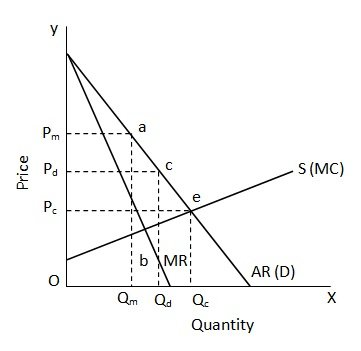Consequences of Price Discrimination

# Consequences of Price Discrimination Assignment Help

Home / Microeconomics Assignment Help / Consequences of Price Discrimination

# Consequences of Price Discrimination

A monopoly firm is able to discriminate between two markets due to differences in their demand elasticity and the different slope of AR and MR curves. The firm equates its MC with MR in each of the two markets. If this was not done, then profits could be increased by transferring a product from low MR market to a higher MR market. With different demand curves in two markets and equating marginal revenue to marginal cost in each market would mean charging a higher price in a market where demand is inelastic and lower price in the market where demand is elastic. This would naturally mean that price discrimination provides higher total revenue to the monopoly compared to the profit maximizing single price simple monopoly.

Another consequence of price discrimination is that output under discriminating monopoly will generally be larger than under a simple single – price monopoly. The equilibrium of a monopoly is at that level of output where MC = MR while for a competitive firm equilibrium output is where MC = MR = AR (or P). since, under monopoly  MR < AR (P), the equilibrium output under monopoly Qm is less than competitive output Qc. reduced output under monopoly also means higher price Pm than the competitive price Pc.A single price monopoly will produce Qm quantity (where MC = MR) and charge a uniform per unit price Pm. now if the firm can sell its output in separate blocks, it would sell OQm output at price Pm. the firm would add to its profits so long as it can sell additional units at a price which is above or equal to MC. Output range Qm to Qc could also be produced and sold at price Pd and further output Qd to Qc could be sold at price OPc. All these expansions in output would add to total profit provided price charged is not uniform at Pc , but it is Pm for output OQm, Pd for output QmQd and Pc for output block Qd to Qc . we can thus separate consumer groups and sell OQm at price Pm. but is willing to buy quantity Qm Qd at price Pd, thereby raising the quantity produced as well as profits of the monopoly firm. If further isolation of consumers could be possible, they can be supplied quantity QdQc at price Pc. This would help in achieving the competitive level of output OQc but this entire output would not be sold at competitive price Pc, but at different prices Pm, Pd and Pc to various isolated groups of customers.

Thus, under discriminating monopoly quantity produced is higher than under simple monopoly and the dead weight loss of monopoly decreases due to increase in both consumers, and producers’ surplus.

### Submit Homework

Submit your homework for a free quote